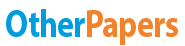# Analysis of Soda Ash

Essay by   •  May 24, 2016  •  Lab Report  •  399 Words (2 Pages)  •  1,269 Views

## Essay Preview: Analysis of Soda Ash

Report this essay
Page 1 of 2

Ryan Hines

Analysis of Soda Ash

Purpose

The purpose of this experiment is to find the percent by mass of sodium carbonate in an unknown sample of soda ash.

Theory

In this experiment we have to find the percent by mass of sodium carbonate in a sample of soda ash. Soda ash is a mixture of ionic compounds including sodium carbonate. We will find this by titrating the sample of soda to the equivalence point, which is when all of when all of the reactants have been reacted. A titration is when you have a known concentration of a solution that is used to determine the concentration of the unknown solution, usually used by a burette dispensing the known solution into a container with the unknown solution.   This will be shown by adding an indicator which will allow us to see when the equivalence point is reached.

The sodium carbonate is basic and will react with the hydrochloric acid. 2HCl + Na2CO3 H2CO3 + 2NaCl. Hydrochloric acid solutions cannot be prepared by very accurately because it cannot be obtained with the correct purity required. Because of this we have to standardize the solution by titrating the sodium hydroxide solution Standardization is the process by determining the exact concentration of a reagent used in a titration. NaOH + HCl H2O + NaCl. Although, it is impossible for us to weigh the NaOH accurately because it absorbs water, so we also have to standardize this. A primary standard, a substance whose purity can be found with a high degree of accuracy, will be used to standardize the NaOH. The primary standard used will be KHP. So this experiment will need three titrations:

1. Standardization of NaOH
2. Standardization of HCl
3. Titration of soda ash

When titrating to the equivalence point we have to use a different indicator because the soda ash is neutral. We will use the indicator containing indigo carmine and methyl orange. This will make the end point a pale bluish-gray color. We will be titrating in the HCl solution.

Results

Unknown sample #3. The average percent by mass of sodium carbonate is 41.2%.

Conclusion

The pH of the soda ash endpoint is 6.68. This makes sense because we added a strong acid to a weak base which will make the solution less basic. The equation that shows this is:

CO3 + HCl H2CO3

...

...